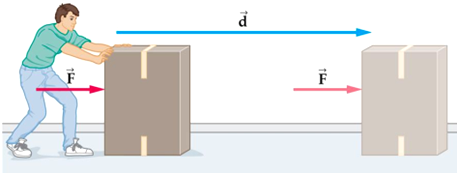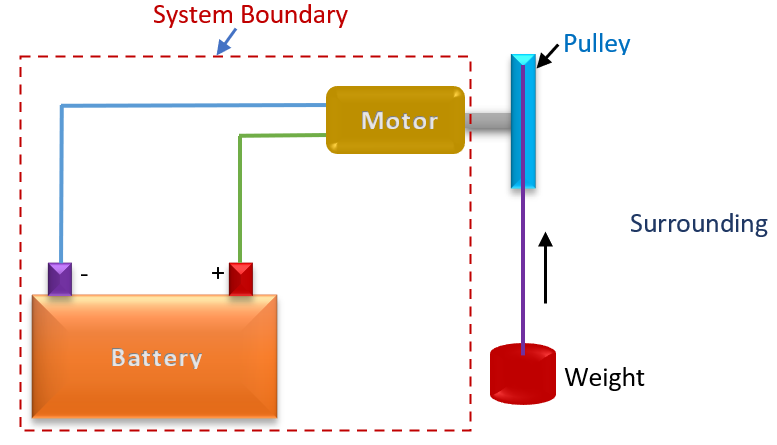### Work, Heat and Energy

Work, Heat and Energy
Work

1. Mechanics Definition
Work is done when the point of application of a force moves in the direction of the force. The amount of work is equal to the product of the force and
i.e. W = F x L
the distance through which the point of application moves in the direction of the force. i.e., work is identified only when a force moves its point of
application through an observable distance.Picture: Mechanical Work
However, when treating thermodynamics from a macroscopic point of view, it is advantageous to define work with the concepts of systems,
properties and processes.

1. Thermodynamic Definition
It is said to be done by a system when the ‘sole effect’ external to the system could be reduced to the raising of a weight. The word ‘sole effect’
indicates that the raising of weight should be the only interaction between the system and surroundings in order to say that there is work interaction
between the system and the surroundings. It is a boundary phenomenon, since it occurs only at the boundary of a systemFigure: Thermodynamic Work
This is illustrated in the figure. The sole effect on things external to the system is then the raising of a weight.
Unit for Work-

• The unit of work is Nm or Joule.

Heat
Heat is defined as the form of energy that is transferred across a boundary by virtue of a temperature difference or gradient. Heat is a form of energy
in transit. It is a boundary phenomenon, since it occurs only at the boundary of a system.

Unit for Heat-

• The unit of heat is Nm or Joule.

Energy
Energy is defined as the capacity to perform work. However, it's important to know that just because energy exists, it's not necessarily available to do work.
Forms of Energy

• It exists in several forms such as heat, kinetic or mechanical energy, light, potential energy, and electrical energy. Other forms of energy may include
• geothermal energy and classification of energy as renewable or nonrenewable.
• Heat or Thermal energy - Energy from the movement of atoms or molecules and is the energy relating to temperature.
• Kinetic Energy - Energy of motion. For example, a swinging pendulum has kinetic energy.
• Potential Energy - Energy due to an object's position. For example, a ball sitting on a table has energy stored in it because gravity acts upon it.
• Mechanical Energy - Is the sum of the kinetic and potential energy of a body.
• Light - Photons are a form of energy.
• Electrical Energy - Energy from the movement of charged particles, such as protons, electrons, or ions.
• Magnetic Energy - Energy resulting from a magnetic field.
• Chemical Energy - Energy released or absorbed during chemical reactions. It is produced by breaking or forming chemical bonds between atoms
• and molecules.
• Nuclear Energy - This is energy from interactions with the protons and neutrons of an atom. Examples are energy released by fission and fusion.